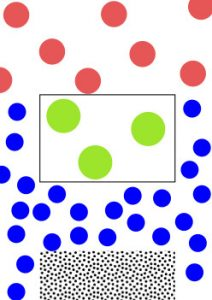# Fluid Density

Matter exists in three common phases:

• solid
• liquid
• gas

A solid has a defined shape and specific volume.

A fluid is described as a body/matter in which the atoms can move/slide relative to each other. Because of this property, when poured into a container, the fluid will take the shape of it. Also, a fluid is perfectly elastic, it resists any applied stress and will return to its original size and shape after the stress is removed.

There are two types of fluids: gases and liquids.

A gas can be compressed and it will fill all the volume within a closed container.

A liquid is a fluid which can not be compressed. As any body on Earth, a liquid is affected by gravitational forces. Due to this, in any container or vase/bowl, a stored liquid will have a horizontal plane surface when in equilibrium.

At the molecular/atom level, a solid has the atoms in fixed position. Depending on the type of solid material, the atoms can be distributed in a very compact form (e.g. metal) or in a loose form (e.g. wood).

The atoms in a liquid are not in fixed position, they can move relatively to each other. The atoms in a liquid are relatively closely packed to each other.

In a gas atoms are separated by distances which are considerably larger than the atom size. Therefore, the forces between atoms are very week and the gas can be easily separated in different parts.Image: Particles density – gas (red), liquid (blue) and solid (green – e.g. wood, black – e.g. metal)

Density is a very common and important property of matter. For a liquid, density is defined as the mass contained in a unit volume. The more precise name for density is volumetric mass density.

The symbol used for density is the Greek letter ρ (rho).

Mathematically, density (ρ) is defined as the ratio between the mass (m) and the volume (V) of a substance/body:

$\begin{equation} \begin{split} \bbox[#FFFF9D]{\rho = \frac{m}{V}} \end{split} \end{equation}$

The unit of measurement for density in SI is kg/m3.

For a particulate substance, having two parameter defined (mass, density or volume), we can calculate the third one:

$\begin{equation*} \begin{split} V &= \frac{m}{\rho}\\ m &= \rho \cdot V \end{split} \end{equation*}$

Example 1. Calculate the mass of the fuel stored inside the tank of a gasoline engine powered vehicle. The fuel tank has a total volume of 55 liters.

First of all we need to know what’s the density of gasoline. It ranges between 720 and 770 kg/m3. For our example we are going to set the density of gasoline at 745 kg/m3.

Also we convert the volume of 55 liters into SI units, which is 0.055 m3.

Now we can calculate the mass of the fuel in the tank.

$m = \rho \cdot V = 745 \cdot 0.055 = 40.975 kg$

This calculation is useful when you need to know the impact of the maximum amount of fuel on the total mass of the vehicle.

Liquids are subjects to volumic thermal expansion, which means that liquids increase their volume if the temperature increases.

Therefore, for a particular liquid, density is not constant when temperatures vary. The dependency of density function of temperature is:

$\begin{equation} \begin{split} \bbox[#FFFF9D]{\rho = \frac{\rho_{T0}}{1+\alpha \cdot \Delta T}} \end{split} \end{equation}$

where:

ρT0 [kg/m3– density at a reference temperature T0
α [1/°C] – thermal expansion coefficient of the liquid at temperature T0
ΔT [°C] – temperature difference

$\Delta T = T – T_0$

where:

T0 [°C] – reference temperature
T [°C] – current temperature

Example 2. The density of water at 20 °C is 998.2071 kg/m3. The thermal expansion coefficient for water is 69·10-6 1/K at 20 °C. What is the density of water at 80 °C ?

First we calculate the temperature difference between our reference temperature (T0 = 20 °C) and the final temperature (T = 80 °C) at which we need to calculate the density.

$\Delta T = 80 – 20 = 60 \text{ } ^\circ C$

Converting the temperature difference in degrees Kelvin, we get:

$\Delta T = 60 + 273.15 = 333.15 \text{ } K$

The density of the water at temperature T = 80 °C will be:

$\rho = \frac{998.2071}{1+ 69 \cdot 10^{-6} \cdot 333.15} = 975.78 \text{ } \frac{kg}{m^3}$

What happens if we put several substances (fluids and solids), with different densities, in the same container ?

The answer is simple. The liquid/solid with the highest density will be at the bottom of the container while the liquid/solid with the lowest density will be at the top of the container. In the image below you can see a glass column containing 4 liquids and 2 solids of different densities.

Surprised by the result ? Let’s have a look at the density of each substance.

 Substance Phase Density [kg/m3] Rubbing alcohol liquid 786 Baby oil liquid 830 Vegetable oil liquid 920 Wax solid 930 Water liquid 1000 Aluminium solid 2700

Note: artificial coloring was added to baby oil and water for better visibility

As we can see the substance with highest density (aluminium) sits at the bottom of the glass column while the substance with lowest density (rubbing alcohol) sits at the top of the glass column.

For any questions or observations regarding this tutorial please use the comment form below.

Don’t forget to Like, Share and Subscribe!